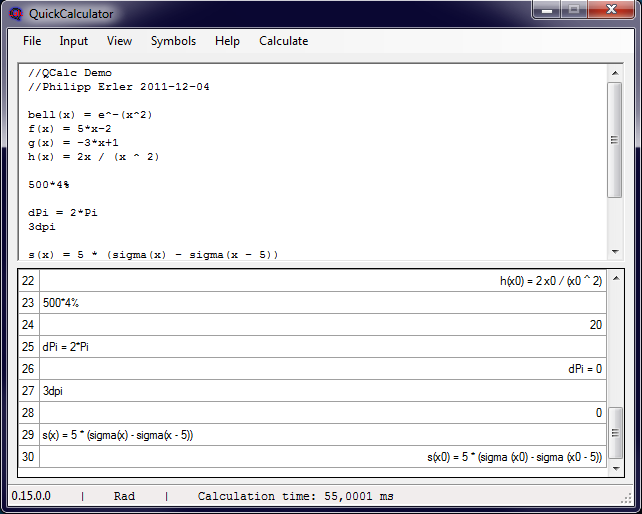# QuickCalculatorThe QuickCalculator is a simple and fast calculation program with scripting and plotting – ideal to quickly visualize e.g. an interpolation algorithm.

Contents

## Why do I need the QuickCalculator?

• The Windows calculator or similar programs don’t offer you enough possibilities?
• You don’t want to learn to use Matlab, Octave, Maple or Mathematica?
• Or you just don’t want to spend money on a calculation program?
• Then the QuickCalculator is what you need!

## What makes the QuickCalculator special?

• It shows your calculations and their results
• It can handle chained operations
• It can handle user defined variables and functions
• It supports user written scripts
• It can draw functions
• No installation is needed
• It is completely free
• It is Open Source

## What can I use the QuickCalculator for?

• Operators
• Addition
• Subtraction
• Multiplication
• Division
• Power
• Radical
• Modulus
• Variables
• Define variables
• Use variables for calculations
• Standard Functions
• Trigonometric functions
• Logarithms
• Sigma / Delta function
• User Functions
• Define functions
• Use functions for calculations
• Draw functions
• Additional
• Round-function
• Answer-variable
• Random-variable
• Hexadecimal, Octal, Binary
• Comparisons
• Bitwise operations
• Scripting
• Control structures
• Script functions for customized startup
• And much more…

## Required Software

.net – Framework 4.0
Mono Runtime (Only needed for Linux and Mac)
7zip